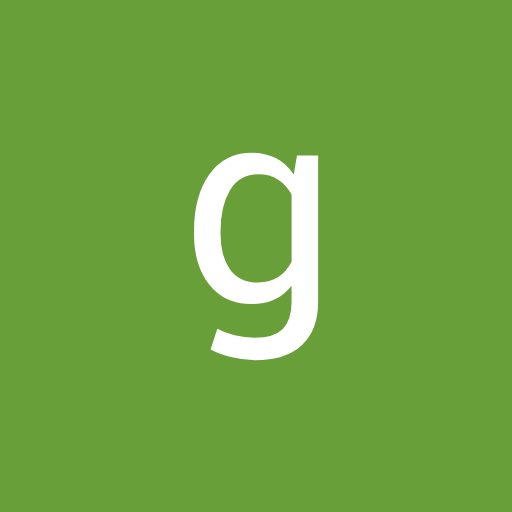# What will come at the place of question mark ?6, 11, 31, 121, 601, ?

A. 3600

B. 3621

C. 3601

D. 3611

### Solution(By Examveda Team)

Logic:
First Term = 6.

Second term = 6*2 -1 = 11.

Third term = 11* 3 - 2 = 31.

Fourth term = 31 *4 - 3 = 124 -3 = 121.

Fifth term = 121*5 - 4 = 601.

Sixth term = 601 *6 = 3606 - 5 = 3601.

1.